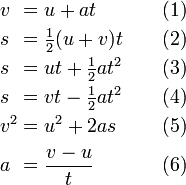# How fast does my starship go?

dividebyxero
I'm trying to figure how fast this ship can reach various destinations in a story I'm working on and I have no background in advance math of any kind (If teachers had offered more interesting scenario questions like this one I might have paid more attention in class, instead they gave me those idiotic "train leaving LA and another leaving NY" sort of questions).

Assume the following:

* The ship uses an Alcubierre warp drive

* It takes 4 weeks to cross the galaxy (100,000 light years in 28 days exactly just for simplicity's sake)

* The ship will accelerate for the first half of its journey, reverse engines, and decelerate for the last half.

My questions are:

- What is its rate of acceleration?

- How fast is it moving at the halfway point of the sample trip above?

- How does one calculate the answer to this sort of question so I could determine the travel time of other trips (from Sol to Polaris for example)?

JaredJames
The kinematics equations will give you what you need.

http://en.wikipedia.org/wiki/Equations_of_motion#Classic_versionYou know u, t and s.

Plug those in and you get the final velocity and the acceleration.

Remember, t and s are half the total values as you are only looking at half the journey. You want t in seconds and s in metres.

Use number 2 to get your final velocity v, and then 1 to get acceleration.

yuiop
Assume the following:

* The ship uses an Alcubierre warp drive

* It takes 4 weeks to cross the galaxy (100,000 light years in 28 days exactly just for simplicity's sake)

* The ship will accelerate for the first half of its journey, reverse engines, and decelerate for the last half.
Newtonian equation are completely inadequate for the speeds you are proposing. For relativistic speeds you should use the equations given here http://www.phys.ncku.edu.tw/mirrors/physicsfaq/Relativity/SR/rocket.html [Broken]

On the face of it using Earth time your ship is travelling at 12,700,000 times the speed of light and even the relativistic equations I gave are inadequate. SR can not handle speeds that are greater than the speed of light. However, if you mean 28 days as measured by the passengers on board the ship (proper time) then your figures *might be* compatible with SR when taking time dilation into account. The acceleration would probably have to be much greater than 1g as used in the link I gave, but this *might* not be a problem for the Alcubierre drive, because the passengers apparently do not feel the acceleration with that form of drive (if it can exist in principle).

Last edited by a moderator:
JaredJames
Newtonian equation are completely inadequate for the speeds you are proposing. For relativistic speeds you should use the equations given here http://www.phys.ncku.edu.tw/mirrors/physicsfaq/Relativity/SR/rocket.html [Broken]

On the face of it using Earth time your ship is travelling at 12,700,000 times the speed of light and even the relativistic equations I gave are inadequate. SR can not handle speeds that are greater than the speed of light. However, if you mean 28 days as measured by the passengers on board the ship (proper time) then your figures *may be* compatible with SR when taking time dilation into account. The acceleration would probably have to be much greater than 1g as used in the link I gave, but this might not a problem for the Alcubierre drive because the passengers apparently do not feel the acceleration with that form of drive (if it can exist in principle).

Alcubbiere drives don't experience time dilation because of the way they are proposed to work.

The equations I gave were simply to give a basic set of values - it's sci-fi, keep it simple and don't try to explain it - especially as the OP has little understanding of the maths required.

Last edited by a moderator:
dividebyxero
Alcubbiere drives don't experience time dilation because of the way they are proposed to work.

The equations I gave were simply to give a basic set of values - it's sci-fi, keep it simple and don't try to explain it - especially as the OP has little understanding of the maths required.

Correct. That's why I mentioned the method of propulsion, I figured that would indicate that time dilation is not meant to be a factor.

In any event, thanks for the replies! :)

dividebyxero
Ok, just want to double check my figures.

* s = The halfway point of the trip (where acceleration will stop) is 50,000 ly or 473036523629040000000 meters

* t = Time to reach that distance is 14 days or 1,209,600 seconds

From those we get:

* v = Final velocity is 782137109175000 meters per second (2,608,928 times the speed of light)

Meaning that the rate of acceleration (a) should be 646608059 meters (or 2.15 light years) per second (per second?)

Furthermore, if I'm reading the suvat equations correctly I should be able to calculate the duration of any other trip by doing line 5 as it is, getting the square root of that result which should end up as v, then using that in: (v - u) / a = t

Is that correct?